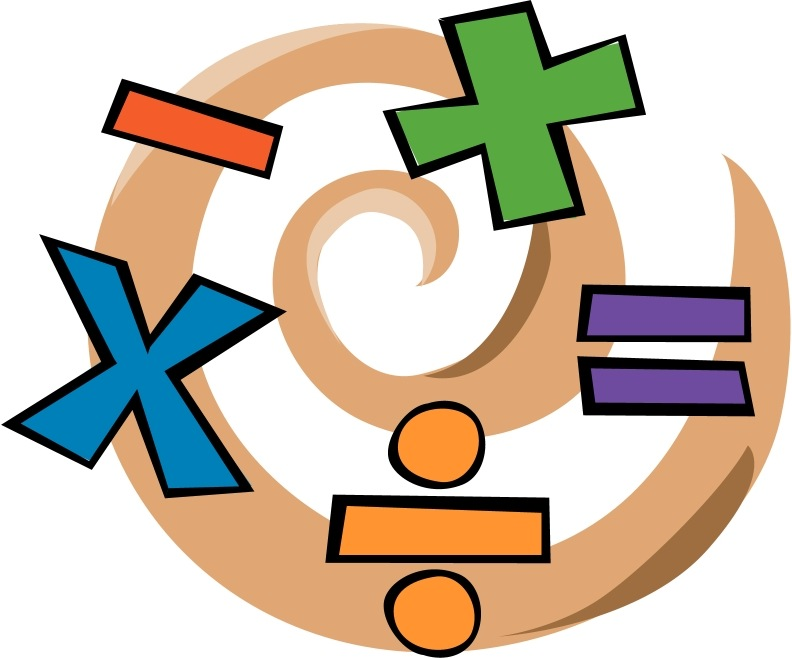Quant Mumbo Jumbo – CAT 2016 – 2IIMThere is a famous declaration in Math that goes thus – “Any prime number greater than 5 can be written in the form 6k + 1″ . This is blather. Pure, unadulterated blather that is a unique product of our system of education. This is not wrong, mind you. It could have been banished easily if it had been. I have never hated something so much for being right.

Why is the 6k + 1 statement odious?

It takes an obvious idea, wraps some jargon-like thing around it and elevates it to axiomatic levels. Let us see why/how. Any natural number can be written in the form 6k + r, where r can take values from 0 to 5. In other words, if we divided a natural number by 6, we could get remainders 0, 1, 2, 3, 4, or 5. Now, a number written as 6k + 5 can also be written as 6k + 6 – 1or 6(k+1) – 1. Or effectively it can be written as 6l – 1. Similarly, 6k + 2 is 6p – 4 and so on.

So, any natural number is either of the form 6k or 6k + 1, 6k + 2, 6k + 3, 6k -2, or 6k – 1. Or, it is of the form 6k, 6k +1, 6k + 2, 6k + 3. A number of the form 6k + 2 will be even and so cannot be prime (except if the number is 2 itself). A number of the form 6k + 3 will be a multiple of 3 and so cannot be prime (except if the number is 3 itself). Obviously, a number of the form 6k cannot be prime.

So, prime numbers can only be of the form 6k + 1. The other, less dramatic, more understandable, simpler, pithier, accurate way of saying this is – Except 2 and 3 no prime numbers are multiples of 2 or 3. But that is not really cool, is it?

And it is not even the case where we are creating a fabulous subset of natural numbers to choose from. One-third of all numbers are of the form 6k + 1.  If we considered remainders with respect to 30, we can say that only numbers of the form 30k + 1, 30k + 7, 30k + 11, or 30k + 13 can be prime. Not particularly pithy, but we are limiting the case to 4/15 of all natural numbers, which is still better than one-third. [Actually, there is a tiny link to Euler’s phi function here, but if we discussed that we would be digressing aggressively]

The freaking converse is not true

All numbers of the form 6k + 1 are not prime. This should be obvious, but our students are fed the first statement so aggressively that they often do not pause to think about the absurdity of the converse. As it turns out, the first few numbers, 5, 7, 11, 13, 17, 19, 23 are all prime. We need to go as far as 25 to find the smallest natural number of the form 6k + 1 that is not prime. And having been fed a diet of pre-packaged absurd pseudo-math one-liners, our students have lost the temperament to try out till the 7th number. It would be hilariously funny if it were not so tragic.

This 6k + 1 reduces math to a mantra that the haves bestow upon the have-nots. This is absurd in the extreme. Euclid’s postulates ( just 5 of them) laid the groundwork for most of Geometry. From taking 5 postulates, and using these to create the entire framework, we have come to the point where we think this 6k + 1 thing is an insight. The marketing men have taken over mathematics.

Dig deeper, find the joy

Math is not to be learnt like this. Stay away from 6k + 1. Go through the proof for the idea that sum of two sides of a triangle is greater than the third, or for the idea that tangent is perpendicular to the radius. These are beautifully constructed. They will put a smile on your face. Chase those.

Rant over.

———-